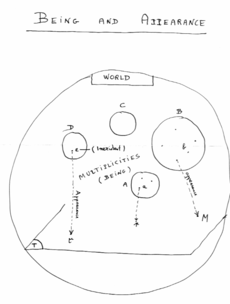# Set Theory Symbols

List of set symbols of set theory and probability.

### Table of set theory symbols

SymbolSymbol NameMeaning / definitionExample
{ }seta collection of elementsA={3,7,9,14}, B={9,14,28}
A Bintersectionobjects that belong to set A and set BA B = {9,14}
A Bunionobjects that belong to set A or set BA B = {3,7,9,14,28}
A Bsubsetsubset has less elements or equal to the set{9,14,28}{9,14,28}
A Bproper subset / strict subsetsubset has less elements than the set{9,14}{9,14,28}
A Bnot subsetleft set not a subset of right set{9,66}{9,14,28}
A Bsupersetset A has more elements or equal to the set B{9,14,28}{9,14,28}
A Bproper superset / strict supersetset A has more elements than set B{9,14,28}{9,14}
A Bnot supersetset A is not a superset of set B{9,14,28}{9,66}
2Apower setall subsets of A
Ƥ (A)power setall subsets of A
A = Bequalityboth sets have the same membersA={3,9,14}, B={3,9,14}, A=B
Accomplementall the objects that do not belong to set A
A \ Brelative complementobjects that belong to A and not to BA={3,9,14},     B={1,2,3}, A-B={9,14}
A - Brelative complementobjects that belong to A and not to BA={3,9,14},     B={1,2,3}, A-B={9,14}
A ∆ Bsymmetric differenceobjects that belong to A or B but not to their intersectionA={3,9,14},     B={1,2,3}, A ∆ B={1,2,9,14}
A Bsymmetric differenceobjects that belong to A or B but not to their intersectionA={3,9,14},     B={1,2,3}, A B={1,2,9,14}
aAelement ofset membershipA={3,9,14}, 3 A
xAnot element ofno set membershipA={3,9,14}, 1 A
(a,b)ordered paircollection of 2 elements
A×Bcartesian productset of all ordered pairs from A and B
|A|cardinalitythe number of elements of set AA={3,9,14}, |A|=3
#Acardinalitythe number of elements of set AA={3,9,14}, #A=3
אalephinfinite cardinality
Øempty setØ = { }C = {Ø}
Uuniversal setset of all possible values
natural numbers set= {1,2,3,4,...}6 ∈ ℕ
integer numbers set= {...-3,-2,-1,0,1,2,3,...}-6 ∈ ℤ
rational numbers set= {x | x=a/b, a,b∈ℕ}2/6 ∈ ℚ
real numbers set= {x | -∞ < x <∞}6.343434 ∈ ℝ
complex numbers set= {z | z=a+bi, -∞<a<∞,      -∞<b<∞}6+2i ∈ ℂ

Statistical symbols